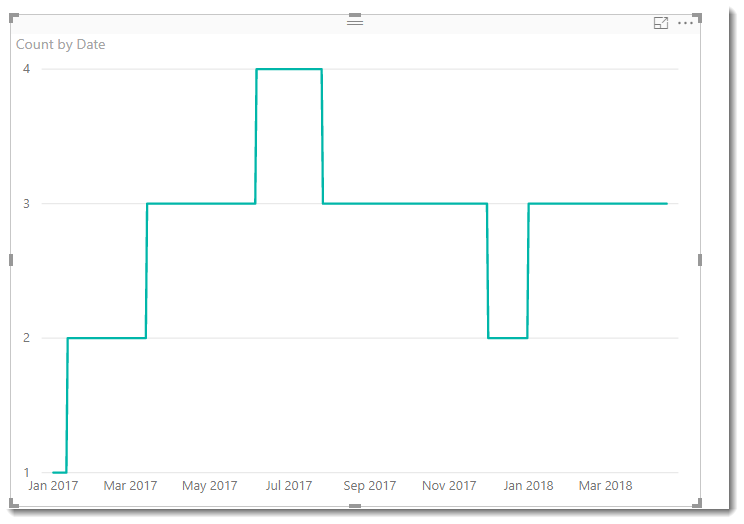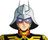cancel
Showing results for
Did you mean:## Number of employee between two dates

Hello DAX Experts,

I'm trying to figure out a formula that calculate the number of employee for each day between two dates. My table looks something like this:I have a calendar table and I need know for each day how many people we have in IT, HR, Fianance, ...etc.

I very much appreacite your help.

2 ACCEPTED SOLUTIONSMicrosoft

This calculated table uses an embedded date table

```Table =
VAR ExpandedTable =
GENERATE(
CALENDAR(DATE(2017,1,1),TODAY()),
FILTER(
'Employees',
[Date]>='Employees'[Start Date] &&
[Date]< IF(ISBLANK('Employees'[End Date]),TODAY(),'Employees'[End Date])
)
)

RETURN
SUMMARIZE(
ExpandedTable,
[Date] ,
"Count",COUNTROWS('Employees')
)          ```and when plotted you get this..Proud to be a Datanaut!Microsoft

Well done on getting it to work with your date table

If you add [Department] to the final SUMMARIZE statement, you can then use this field as a filter

```RETURN
SUMMARIZE(
ExpandedTable,        [Date] ,         [Department] ,
"Count",COUNTROWS('Employees')
)    ```

Proud to be a Datanaut!

8 REPLIES 8Helper I

I suppose you can add a calculate column on this table.

= COUNTROWS(FILTER('Employee'), [JoinDate] >=  [Start Date] && [JoinDate] <= [End Date] && [Employee] = [Employee] && [Department] = [Department])Microsoft

This calculated table uses an embedded date table

```Table =
VAR ExpandedTable =
GENERATE(
CALENDAR(DATE(2017,1,1),TODAY()),
FILTER(
'Employees',
[Date]>='Employees'[Start Date] &&
[Date]< IF(ISBLANK('Employees'[End Date]),TODAY(),'Employees'[End Date])
)
)

RETURN
SUMMARIZE(
ExpandedTable,
[Date] ,
"Count",COUNTROWS('Employees')
)          ```and when plotted you get this..Proud to be a Datanaut!Anonymous
Not applicable

Hi,

By expanding it can quickly become a large table if the dataset (like mine) consist of approx 50.000 rows each year for 5+ years.
Is it possible to get the same output using a measure?

Appreciate thoughts around this approach.Anonymous
Not applicable

Hi,

I believe that I have the solution for how to create this as a measure. See code below

``````Count =
VAR _EndOfMonth =
SELECTEDVALUE( DateTable[EndOfMonth] ) /*Assuming a custom table with a column "EndOfMonth" has been created*/
RETURN
CALCULATE(
DISTINCTCOUNT( Employees[Employee] ),
Filter(
Employees,
Employees[Start Date] <= _EndOfMonth
&& Employees[End Date] >= _EndOfMonth //Assuming no blanks in End Date
)
)``````Hello @Phil_Seamark,

I tried your method but unfornatluty I didn't get the same reults.

I used your method on the calendar table that I have and it worked great except I cannot filter by a department. I understand that I can create a function for department but It'll take a lot to calculate since I have many deprtment. Is there a simpler way?

Thanks.Microsoft

Well done on getting it to work with your date table

If you add [Department] to the final SUMMARIZE statement, you can then use this field as a filter

```RETURN
SUMMARIZE(
ExpandedTable,        [Date] ,         [Department] ,
"Count",COUNTROWS('Employees')
)    ```

Proud to be a Datanaut!Anonymous
Not applicable

I have been trying to figure this out FOREVER. Thank you so much!Thank you, @Phil_Seamark. It works great.

@KeithChu I'll try your formula and let you know.

Thank you both for your contibrution.

BR